# Straight Lines Class 11

In straight lines class 11, the basic concepts of lines such as slopes, angle between two lines, various forms of lines, the distance between lines are given in detail. Now, let us take a look of the straight line class 11 concepts one by one. We are providing here the notes for important topics with formulas for the students so that they can learn from here and prepare for exams. These notes will be of great help for students to score well. Read the complete article for more information.

What is a Straight Line?

A straight line is defined as a line traced by a point travelling in a constant direction with zero curvature. In other words, the shortest distance between two points is called a straight line.

## Slope of a Line

Tan θ is called the slope or gradient of line l if θ is the inclination of a line l. The slope of a line whose inclination is not equal to 900. It is denoted by ‘m’.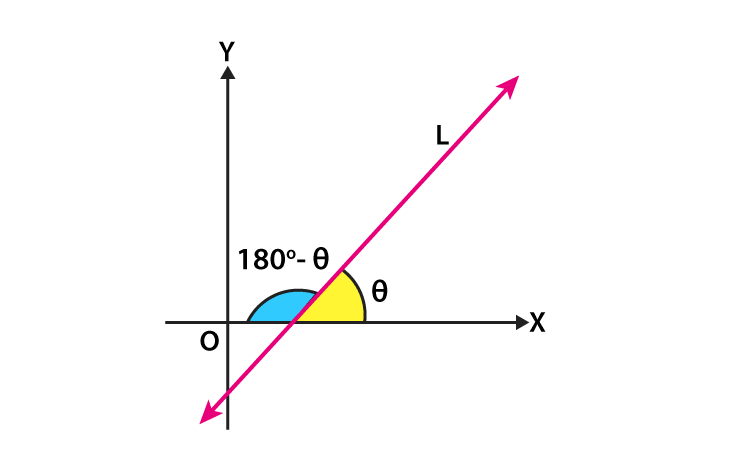Thus, m = tan θ, θ ≠ 90°

If the slope of the y-axis is not defined, it is observed that the slope of the x-axis is zero.

## Angle Between Two Lines

Let’s take the two non-vertical lines L1 and L2 with slopes m1 and m2, respectively where α1 and α2are the inclinations of lines L1 and L2, respectively. Then the slope of the lines m1 and m2 is given as

m1 = tanα1 and m2= tanα2

We know that, when two lines intersect each other, it makes two pairs of vertically opposite angles such that the sum of any two adjacent angles is 180° from the property. Assume that θ and φ be the adjacent angles between the lines L1 and L2. Then

θ = α2– α1 and α1, α2≠ 90°.

Therefore,

tan θ = tan(α21) =  (tan α2 – tan α1)/(1 + tan α1 tan α2)

tan θ = (m2-m1)/(1+m1m2)

Since 1+m1m2 ≠ 0 and φ = 180° – θ, so;

tan φ = tan (180° – θ ) = -tan θ = -(m2-m1)/(1+m1m2)

Case 1: If (m2-m1)/(1+m1m2) is positive, then tan θ will be positive and tan φ will be negative, which means that θ will be acute and φ will be obtuse.

Case 2: If (m2-m1)/(1+m1m2) is negative, then tan θ will be negative and tan φ will be positive, that means that θ will be obtuse and φ will be acute.

Thus, the acute angle between the lines L1 and L2 with slopes m1 and m2 respectively is given by

$$\begin{array}{l}\tan \theta = \left | \frac{m_{2}-m_{1}}{1+m_{1}m_{2}} \right |\end{array}$$

Where, 1 + m1m2 ≠ 0

Then the obtuse angle can be found by using φ =1800– θ.

## Various Forms of Equations of Line

The various forms of the equation of the line covered in straight line class 11 are as follows

### Slope – Point Form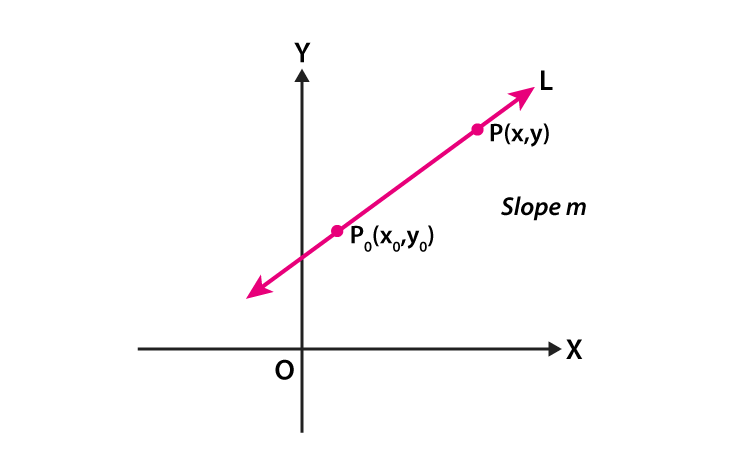Assume that P0(x0, y0) is a fixed point on a non-vertical line L, whose slope is m. Consider that P (x, y) be an arbitrary point on L then the point (x, y) lies on the line with slope m through the fixed point (x0, y0), if and only if, its coordinates satisfy the following equation

y – y0 = m (x – x0)

### Two – Point Form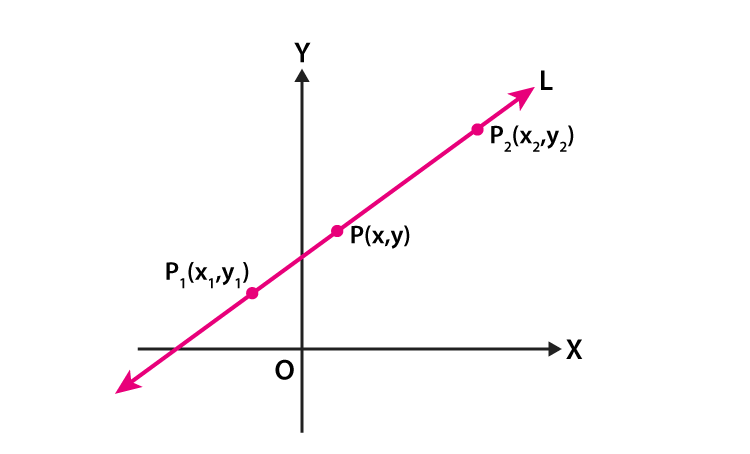Let us take the line L passes through two given points P1(x1, y1) and P2(x2, y2) and P (x, y) be a general point on L. Then the three points P1, P2 and P are collinear, therefore, it becomes

The slope of P1P = The slope of P1P2

$$\begin{array}{l}\frac{y-y_{1}}{x-x_{1}}=\frac{y_{2}-y_{1}}{x_{2}-x_{1}}\end{array}$$
(or)

$$\begin{array}{l}y-y_{1}=\frac{y_{2}-y_{1}}{x_{2}-x_{1}}(x-x_{1})\end{array}$$

Thus, the equation of the line passing through the given points (x1, y1) and (x2, y2) is defined by

$$\begin{array}{l}y-y_{1}=\frac{y_{2}-y_{1}}{x_{2}-x_{1}}(x-x_{1})\end{array}$$

### Slope-Intercept Form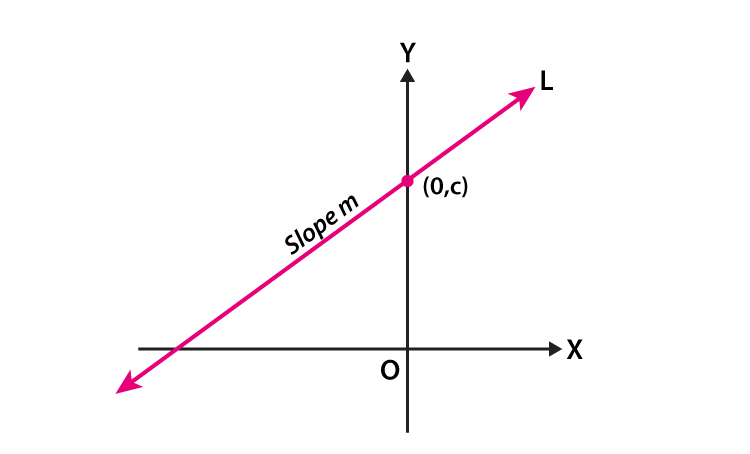Assume that a line L with slope m cuts the y-axis at a distance c from the origin where the distance c is called the y-intercept of the line L. Therefore, the coordinates of the point where the line meets the y-axis are (0, c). So, the line L has slope m and passes through a fixed point (0, c). Thus, from the slope – point form, the equation of the line L is

y – c =m( x -0 )

Therefore, the point (x, y) on the line with slope m and y-intercept c lies on the line if and only if

y = m x +c

Note that the value of c will be positive or negative according to the intercept is made on the positive or negative side of the y-axis, respectively.

### Intercept Form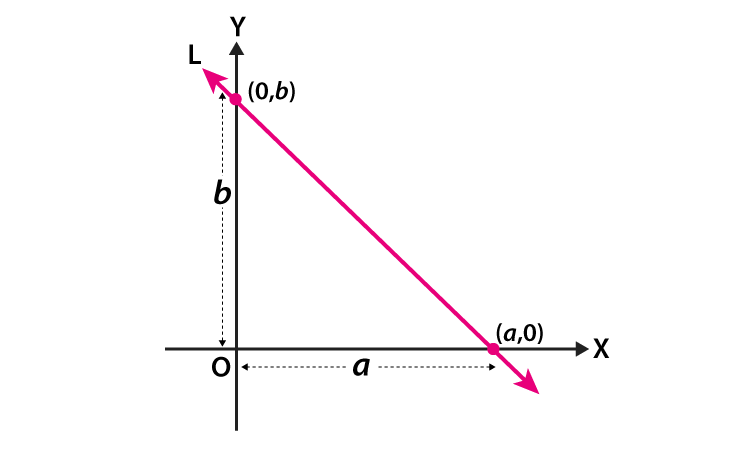Consider a line L that makes x-intercept and y-intercept b on the axes. So that, L meets x-axis at the point (a, 0) and y-axis at the point (0, b)

From the two-point form of the equation of the line, we get

$$\begin{array}{l}y-0 = \frac{b-0}{0-a}(x-a)\end{array}$$

Therefore, it becomes

$$\begin{array}{l}\frac{x}{a}+\frac{y}{b}=1\end{array}$$

Thus, the equation of the line having the intercepts a and b on x-and y-axis respectively is given by

$$\begin{array}{l}\frac{x}{a}+\frac{y}{b}=1\end{array}$$

## Distance of a Point From a Line

Let, the perpendicular distance (d) of a line Ax + By+ C = 0 from a point (x1, y1) is defined by

$$\begin{array}{l}d=\frac{\left | Ax_{1}+By_{1}+C \right |}{\sqrt{A^{2}+B^{2}}}\end{array}$$

## Distance Between Two Parallel Lines

The distance d between the two parallel lines say, y= m x+ c1 and y = m x + c2 is given by

$$\begin{array}{l}d=\frac{\left | C_{1}-C_{2} \right |}{\sqrt{1+m^{2}}}\end{array}$$

Consider the general form of the line, i.e., Ax + By + C1= 0 and Ax + By + C2=0

$$\begin{array}{l}d=\frac{\left | c_{1}-c_{2} \right |}{\sqrt{A^{2}+B^{2}}}\end{array}$$

## Sample Problem

Go through the straight line class 11 problem provided here.

Question: If the three lines 2x + y – 3 =0 , 5x + ky – 3 = 0 and 3x – y – 2 = 0 are concurrent then find the Value of k.

Solution: If three lines are said to be concurrent when they pass through a common point. It means that the point of intersection of two lines lies on the third line.

Given equation :

2x + y – 3 =0 ……(1)

5x + ky – 3 = 0 ……(2)

3x – y – 2 = 0 ……..(3)

By solving the line equation (1) and (3) using cross multiplication method,

$$\begin{array}{l}\frac{x}{-2-3}=\frac{y}{-9+4}=\frac{1}{-2-3}\end{array}$$
(or)

x = 1 and y = 1

Therefore, the point of intersection of two lines is given as (1, 1). By substituting the point (1, 1) in equation (2), we get

5.1 + k .1 – 3 = 0 or k = – 2.

Therefore, the value of k is -2.

### Straight Lines – Important JEE Main QuestionsRegister with BYJU’S – The Learning App for more information on maths-related articles and also watch interactive videos to learn with ease.

Test your knowledge on Straight Lines Class 11